Mobile QR Code1. (Radiation Engineering Dept. National Center for Radiation Research and Technology (NCRRT), Egyptian Atomic Energy Authority (EAEA), Cairo, Egypt)

VCSEL, rectangular pulse, transfer function, convolution theorem, LED mode

## I. INTRODUCTION

The Vertical Cavity Surface Emitting Laser (VCSEL) is an important class of devices that matched well the revolution in optical fiber communication systems, since it has different advantages over other light sources like cost efficiency, high speed and low power consumption and many other features (1-3). VCSELs are also utilized as optical sources for photonic integrated circuits applications due to their low consumption of power (4).

Vertical cavity surface emitting laser (VCSEL) is efficiently utilized for short pulse generation among other light sources due to its high speed response. The concept of gain switching under different injection input pulses are utilized effectively to achieve a maximum generation of power with minimum input pulse width; this is an essential factor for getting long distance and high speed optical communication systems (5). These devices are used in three-dimensional (3D) sensing, such as in gesture and face recognition, eye movement tracking; also they are applied in 3D photography and autofocusing (6). Recently, VCSEL are used in smartphones due to the narrow spectral width (7).

An efficient coupling with different profiles of optical fiber waveguides can be achieved via the VCSELs because it has a narrower line width, lower numerical aperture, and smaller spot size (8). There are many ways to get a high gain and a higher modulation bandwidth. To increase the modulation bandwidth and gain, a short size of cavity and an oxide layer are preferred to get high resonance frequency and high confinement performance (9). An alternative method is to use multiple oxide layers to reduce the parasitic effect resulted from the increasing thickness of the oxidized layer (10,11).

In addition to the above, there are other challenges facing these devices. For example, it is required to solve the limitation of modulation speed of VCSEL resulting at a high temperature. Also, power consumption for both data centers and High Performance Computers (HPCs), where large power consumption increases the cost. Large power consumption will limit the stability of VCSELs (12). In the future, VCSEL is still the crucial Laser source in high speed data communication and optical interconnect systems. The improvement of bandwidth and bit rate of the next generation VCSEL at high temperature is possible. Also, a vertical and direct integration between VCSEL and PIN-PD will create transceivers in optical communication links. This can be well applied in future data communication (13).

The goal of this paper is to analyze the effects of an injected input rectangular pulse with different widths on the transient behavior of a Vertical Cavity Surface Emitting Laser (VCSEL). This model is useful to investigate the different factors that control the speed of that device at different regions of the output response to be utilized in optical communication systems.

## II. THEORETICAL ANALYSIS

### 1. Structure and Operation of the Device

Vertical Cavity Surface Emitting Laser (VCSEL) as shown in Fig. 1 consists of an oxide layer, an active layer, two electrodes and two Distributed Bragg Reflectors (DBRs). A pair of corrugated materials with high and low refractive index is used to form DBR region, the aim of this scheme is to achieve total reflection in a certain range of wavelength. To get a circular shape emitted beam, the oxide layer is designed against the active layer to achieve that goal. Once the emitted light became circular in shape, this will ease the VCSEL to be efficient coupled with photonic circuits and optical fiber waveguides.

Fig. 1. Schematic Structure of a Vertical Cavity Surface Emitting Laser (VCSEL).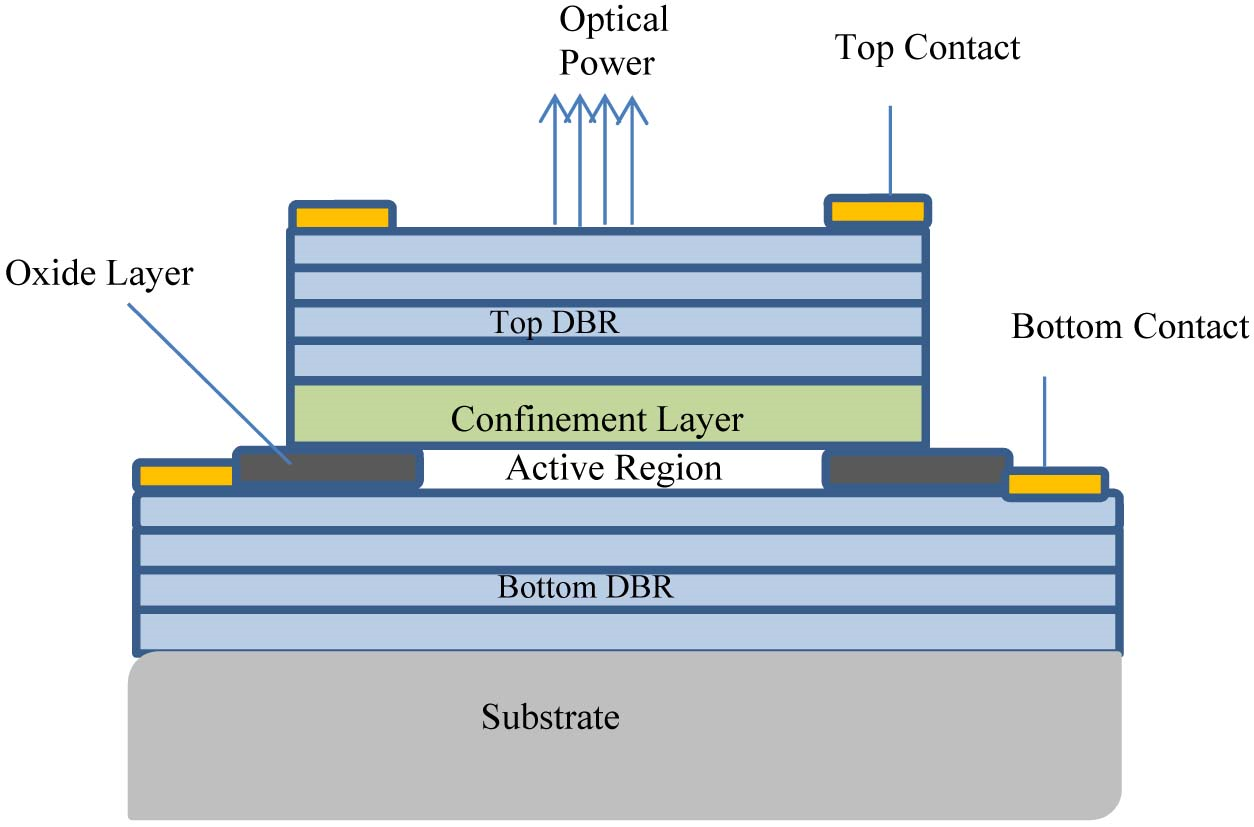### 2. Dynamic Behavior

The transfer function of VCSEL can be written in the s domain as (14,15).

##### (1)
$$M\left(s\right)=A~ \frac{{\omega _{r}}^{2}}{s^{2}+s\gamma +{\omega _{r}}^{2}}$$

where $A=\eta \frac{h~ c}{\lambda q}$, $\eta$ is the conversion efficiency, $c$ is the light velocity, $\lambda$ is the light wavelength, $q$ is the unit charge and $h$ is the blank’s constant.

The resonance frequency $\omega _{r}$ and the damping parameter $\gamma$ are described as:

##### (2)
$$\omega _{r}=\sqrt{\frac{1}{2\pi }\frac{v_{g}\eta _{i}a}{qV_{m}}\left(I- I_{th}\right)\,\,\,}and\,\,\,\gamma =\frac{1}{\tau }+K{\omega _{r}}^{2}$$

where $\eta _{i}$ is the injection efficiency, $\tau$ is the carrier lifetime, $v_{g}$ is the photon group velocity, $g$ is the gain coefficient, $V_{m}$ is the cavity volume, \textit{K} is the damping factor and ($I- I_{th}$) is the above threshold injection current.

When the VCSEL is derived by a rectangular pulse signal with a width of b can be written in the form

##### (3)
$$\Pi \left(t\right)=\left\{\begin{array}{ll} 1 & 0\leq t\leq b\\ 0 & t>b \end{array}\right.$$

In terms of a unit step signal

##### (4)
$$\Pi \left(t\right)=U\left(t\right)- U\left(t- b\right)$$

where

##### (5)
$U\left(t\right)=\left\{\begin{array}{ll} 1 & t\geq 0\\ 0 & \textit{elsewhere} \end{array}\right.$

The input rectangular pulse signal of width b can be expressed in the s domain as

##### (6)
$$\Pi \left(s\right)=L\left(\Pi \left(t\right)\right)=\frac{1}{s}\left(1- e^{- bs}\right)$$

The optical output power can be expressed in the s domain as follow

##### (7)
$$P\left(s\right)=\Pi \left(s\right)M\left(s\right)=\frac{A}{s}\left(1- e^{- bs}\right)\frac{{\omega _{r}}^{2}}{s^{2}+s\gamma +{\omega _{r}}^{2}}$$

By setting the inverse Laplace transform for Eq. (7) to get the time domain output power $P\left(t\right)$

##### (8)
$$P\left(t\right)=A\left[\omega _{r}\,U\left(b- t\right)+\frac{\omega _{r}e^{- {\omega _{r}}t}}{\gamma }- \frac{{\omega _{r}}^{2}e^{- t}}{\gamma }- \frac{\omega _{r}\left(\omega _{r}e^{\left(b- t\right)}+e^{{\omega _{r}}\left(1- t\right)}\right)U\left(b- t\right)}{\gamma }\right]$$

The output derivative as a measure of the speed at which the optical power output is building out can be get as

##### (9)
$$S\left(t\right)=\frac{d}{dt}P\left(t\right)$$

So

##### (10)
\begin{align*} \begin{array}{l} S\left(t\right)=\frac{\omega _{r}}{\gamma }A\left\{\delta \left(t- b\right)- \omega _{r}e^{- {\omega _{r}}t}+\omega _{r}~ e^{- t}- \right.\\ \left.\omega _{r}\left(e^{\left(b- t\right)}+e^{{\omega _{r}}\left(b- t\right)}\right)U\left(b- t\right)+\left(e^{\left(b- t\right)}+e^{{\omega _{r}}\left(b- t\right)}\right)\delta \left(b- t\right)\right\} \end{array} \end{align*}

If the VCSEL operates in the LED mode

##### (11)
$$P(t)=\frac{A}{\gamma}\left\{U(b-t)-U(b-t) e^{-\gamma(t-b)}-e^{-\gamma t}\right\}$$

##### (12)
$$S(t)=\frac{1}{\gamma}\left\{-\delta(t-b)+\delta(t-b) e^{-\gamma(b-t)}+\gamma U(t-b) e^{-\gamma(t-b)}\right\}$$

## III. RESULTS AND DISCUSSIONS

The input signal to the considered device is assumed to be a rectangular pulse of 10 mA amplitude and different widths from 4 ns to 8 ns. The aim of that is to depict the temporal response of VCSEL to the incoming digital optical data via an optical communication system. The transient behavior of VCSEL at different values of resonance frequency is shown in Fig. 2. The device under investigation is derived by a rectangular pulse of width 4 ns. It is clear that the output response with largest values of resonance frequency reaches the largest values of output power. At the end of the pulse, the output response get down gradually to the lowest state, the output with the highest resonance frequency is the later arrival to this lowest state. The output derivative as a measure of speed of the output changes is shown in Fig. 3. The solid line curve represents the speed of response at a resonance frequency of 5 GHz and the dotted line curve corresponds to the speed of response at a resonance frequency of 4 GHz, while the dashed line curve corresponds to the speed of response at a resonance frequency of 3 GHz. It is clear from the figure; the speed of the output is faster for the larger values of resonance frequency. After that, the speed of the output is faster for the lower values of resonance frequency due to the larger time needed from high current state value to the low current state value. There is a steady state time response nearly from 3 ns to 4 ns, so there is no significant change of speed in this time slot. After that the device is back to its previous behavior where the speed of the output is faster for the lower values of resonance frequency due to the same reason.

Fig. 2. The time response of output optical power at different values resonant frequency and b = 4 ns.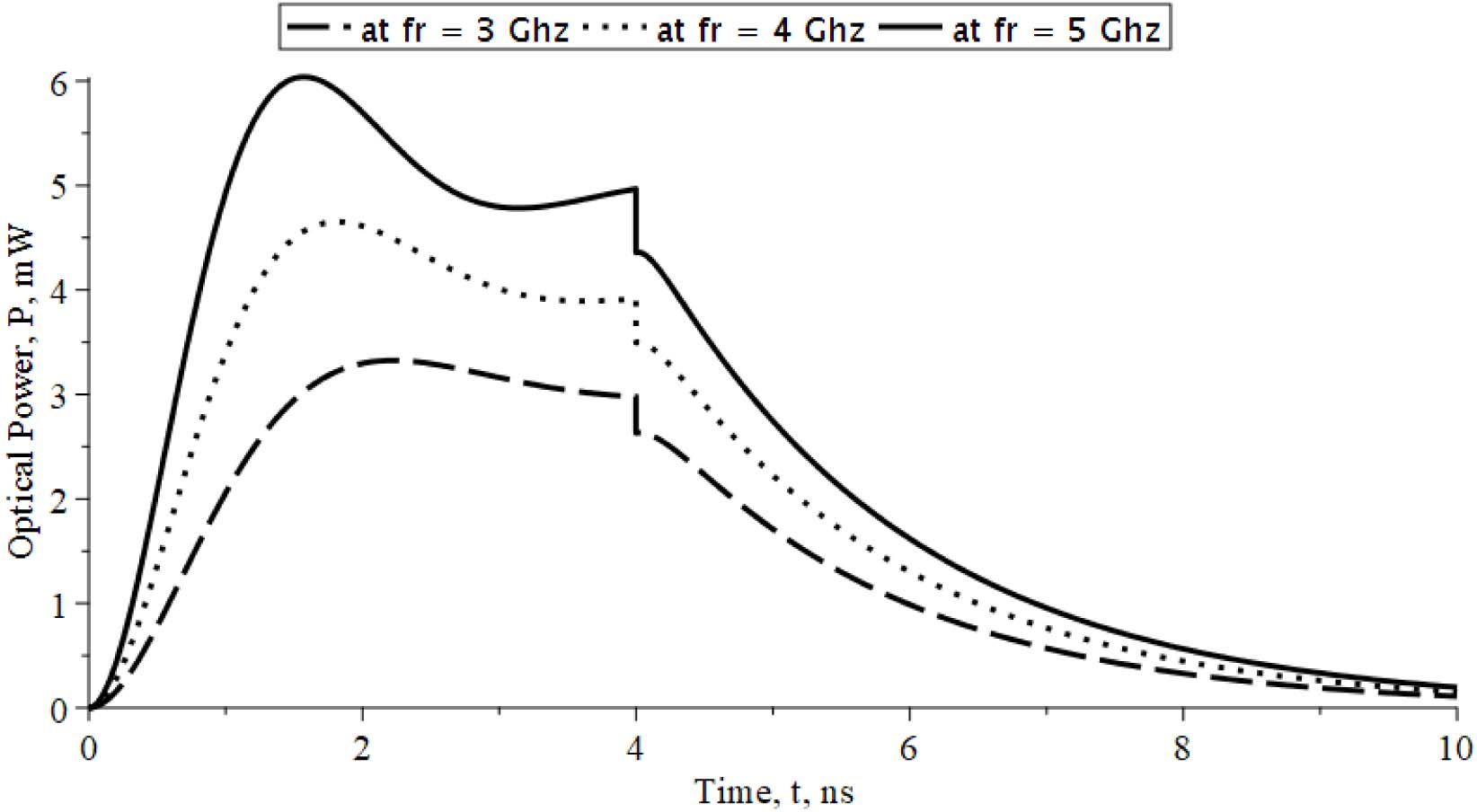Fig. 3. The output derivative of output optical power against time at different values of resonant frequency and b = 4 ns.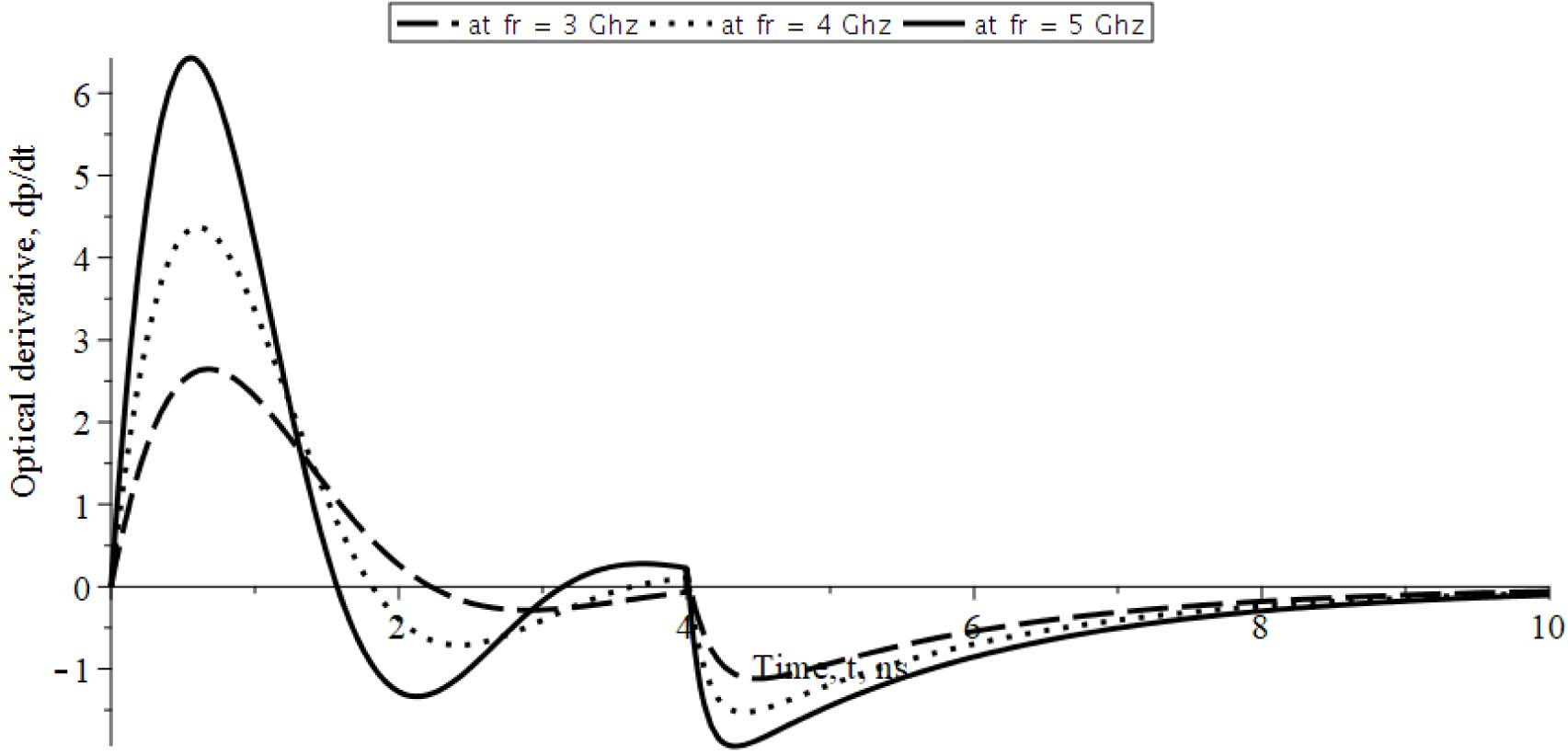Fig. 4. The time response of output optical power at different values of resonant frequency and b = 6 ns.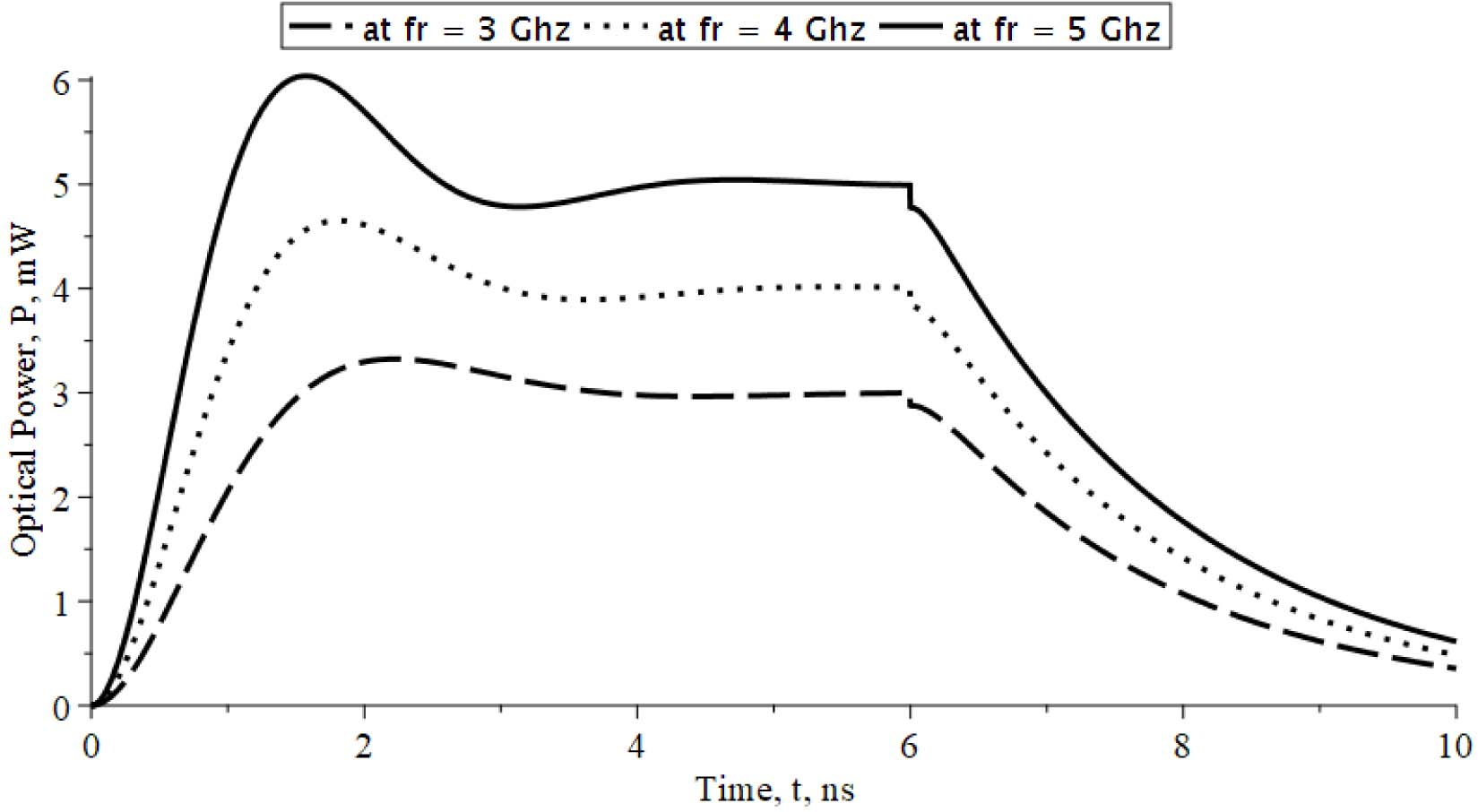When the input pulse is widening from 4 ns to 6 ns, the transient behavior of a VCSEL at different values of resonance frequency and pulse width of 6 ns is shown in Fig. 4. From this set of curves, the transient response gets the chance to appear its steady state behavior. Also, the device takes nearly 2 ns to reach to this steady state value. At the end of the input pulse duration, the device begins to turn off from high state to the low state; the first arrival to the off state is the device with lower values of resonance frequency. The output derivative as a speed behavior of the output changes is shown in Fig. 5. The built-up speed of the output depends on the region of operation, the speed around the first peak power region is faster for the larger resonance frequency, while it is faster for the lower resonance frequency around the first minimum power region. The speed is fixed in the steady state region, while it backs again like the first minimum power region to prepare the device to the off state.

Fig. 5. The output derivative of output optical power against time at different values of resonant frequency and b = 6 ns.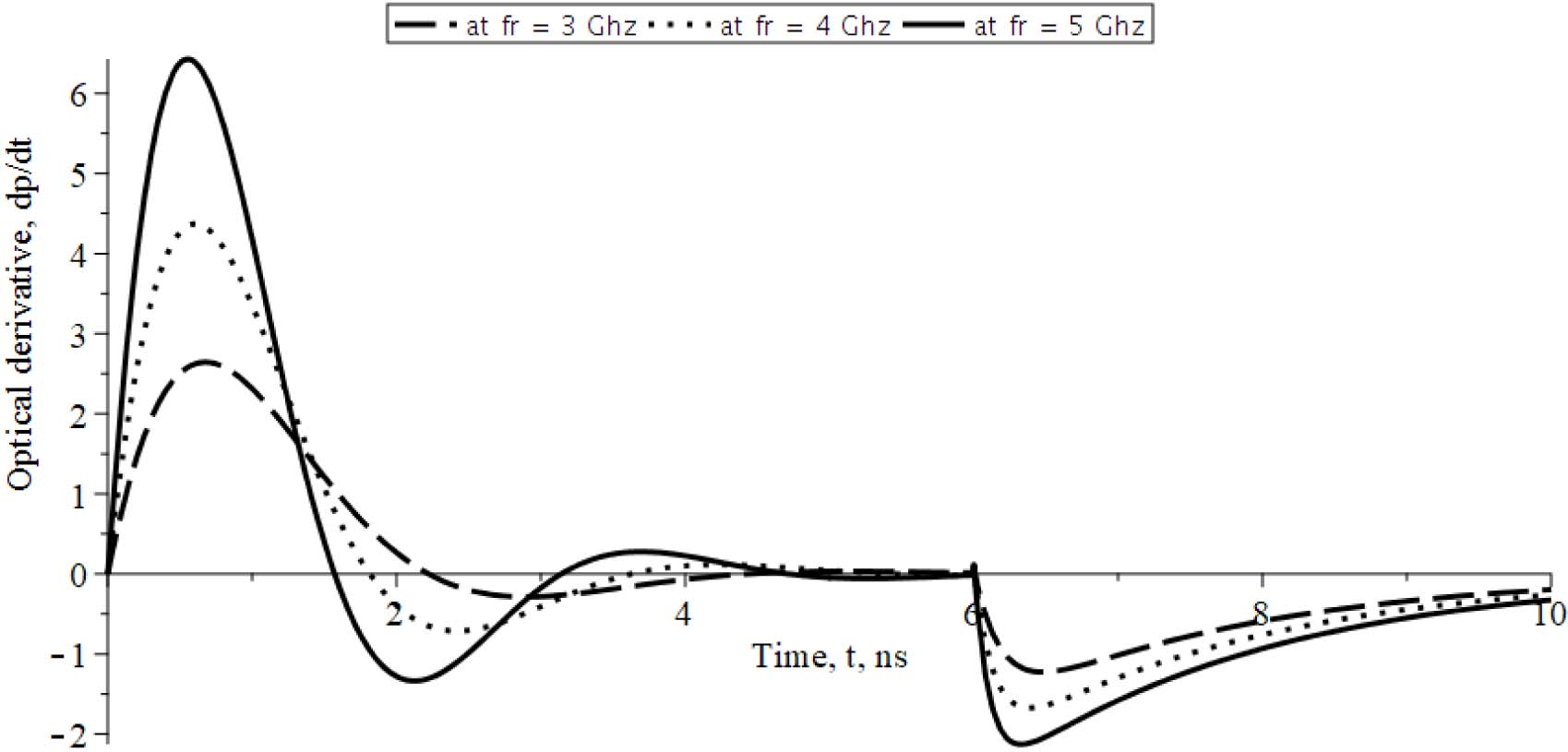Fig. 6. The time response of output optical power at different values of resonant frequency and b = 8 ns.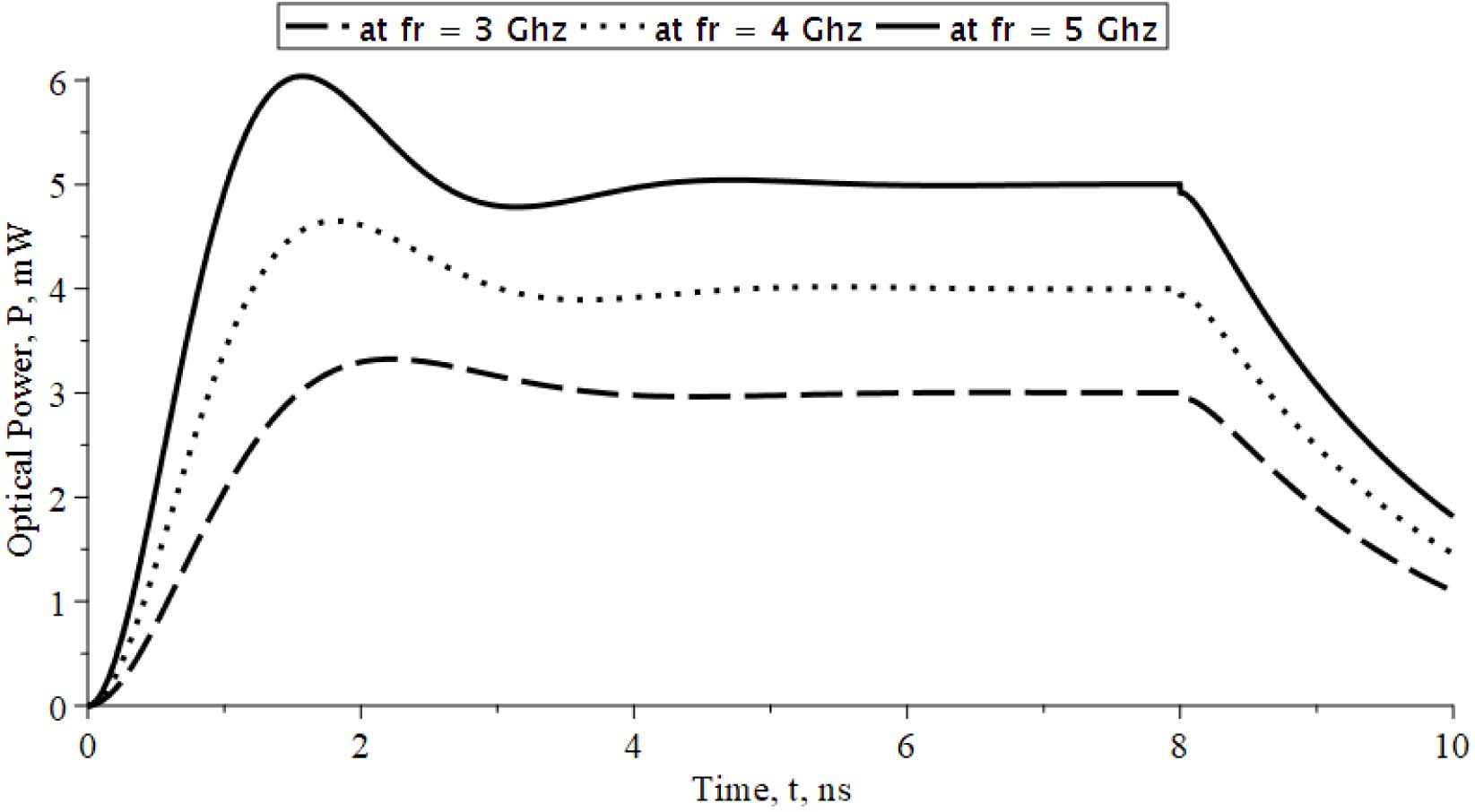The transient behavior of VCSEL at different values of resonance frequency and the pulse width is 8 ns is shown in Fig. 6, From the figure, a laser peak power of 6 mW at 1.6 ns pulse width is obtained when the resonance frequency is fixed at 5 GHz, also the laser peak power of 4.5 mW at 1.76 ns pulse width is obtained when the resonance frequency is settled at 4 GHz, while the laser peak power of 3.1 mW at 1.96 ns pulse width is obtained when the resonance frequency is settled at 3 GHz. These are very important evaluated factors to be utilized in long haul and high speed optical data systems. From 2 ns to 8 ns of the pulse width, the device exhibits a steady state region with a definite value depends on the resonance frequency where it increases with the increase of resonance frequency.

The speed of the device to the different responses is varied from region to another within the time of the input pulse and also after the end of its time period as illustrated in Fig. 7. From the initial time to the end of the first peak power, the device is faster for the larger values of resonance frequency. Unlike the first peak power region is the first bottom power region where the device is faster for the lower values of resonance frequency. After that device speed is stable until the end of the pulse width, after that the device speed is back with a behavior like to the region of bottom power region to make the device off.

Fig. 7. The output derivative of output optical power against time at different values of resonant frequency and b = 8 ns.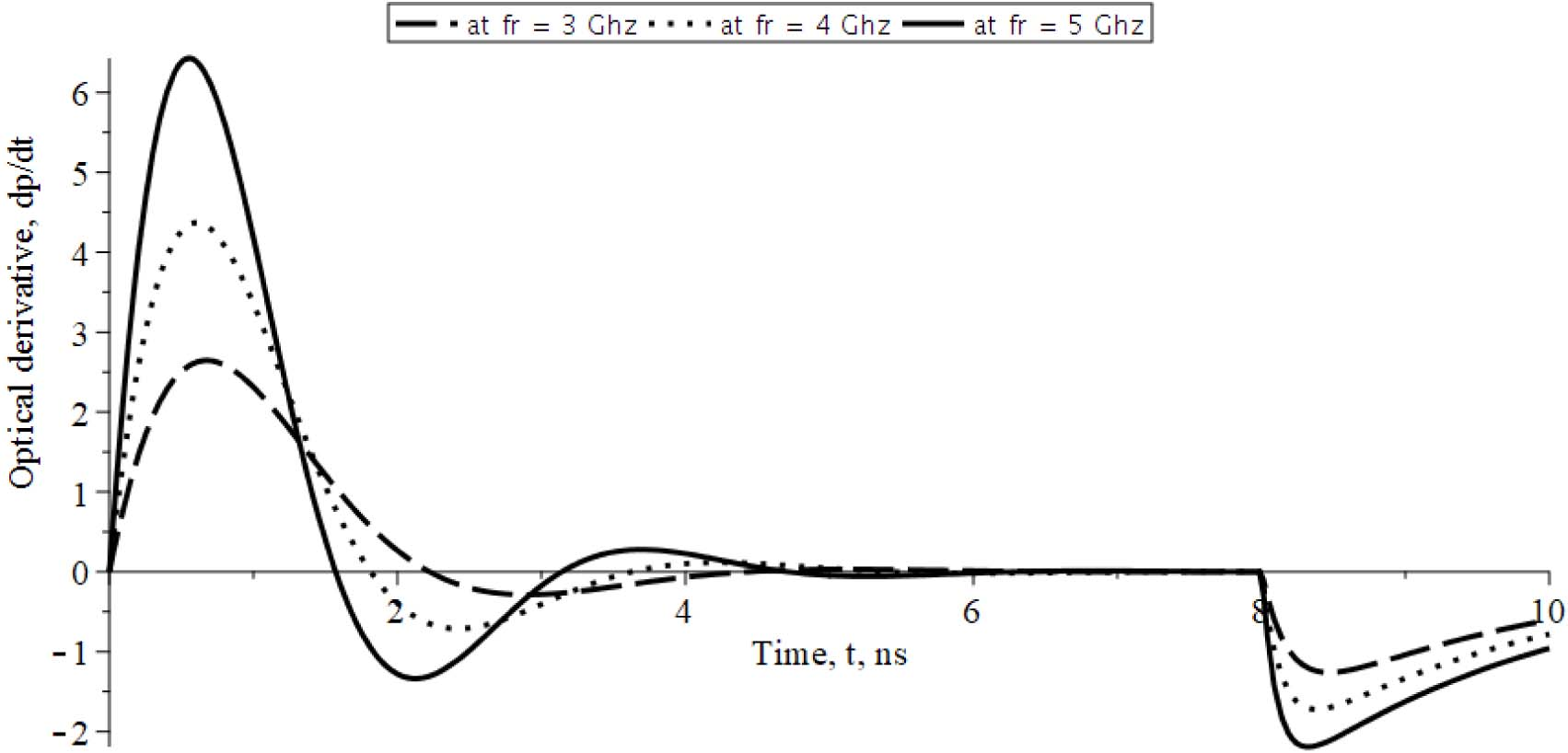Fig. 8. The time response of output optical power at different values of damping frequency.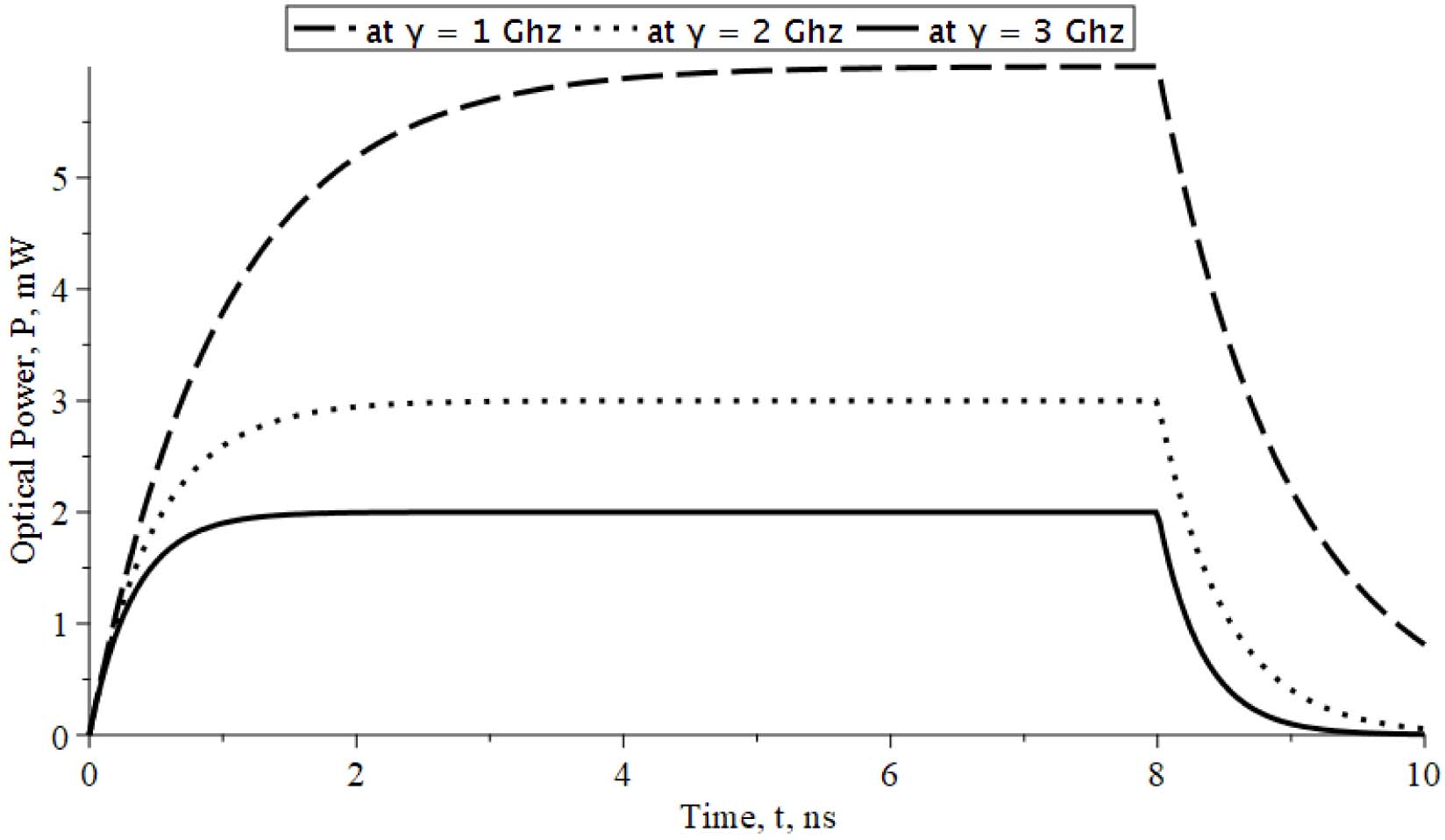Fig. 9. The output derivative of output optical power against time at different values of damping frequency.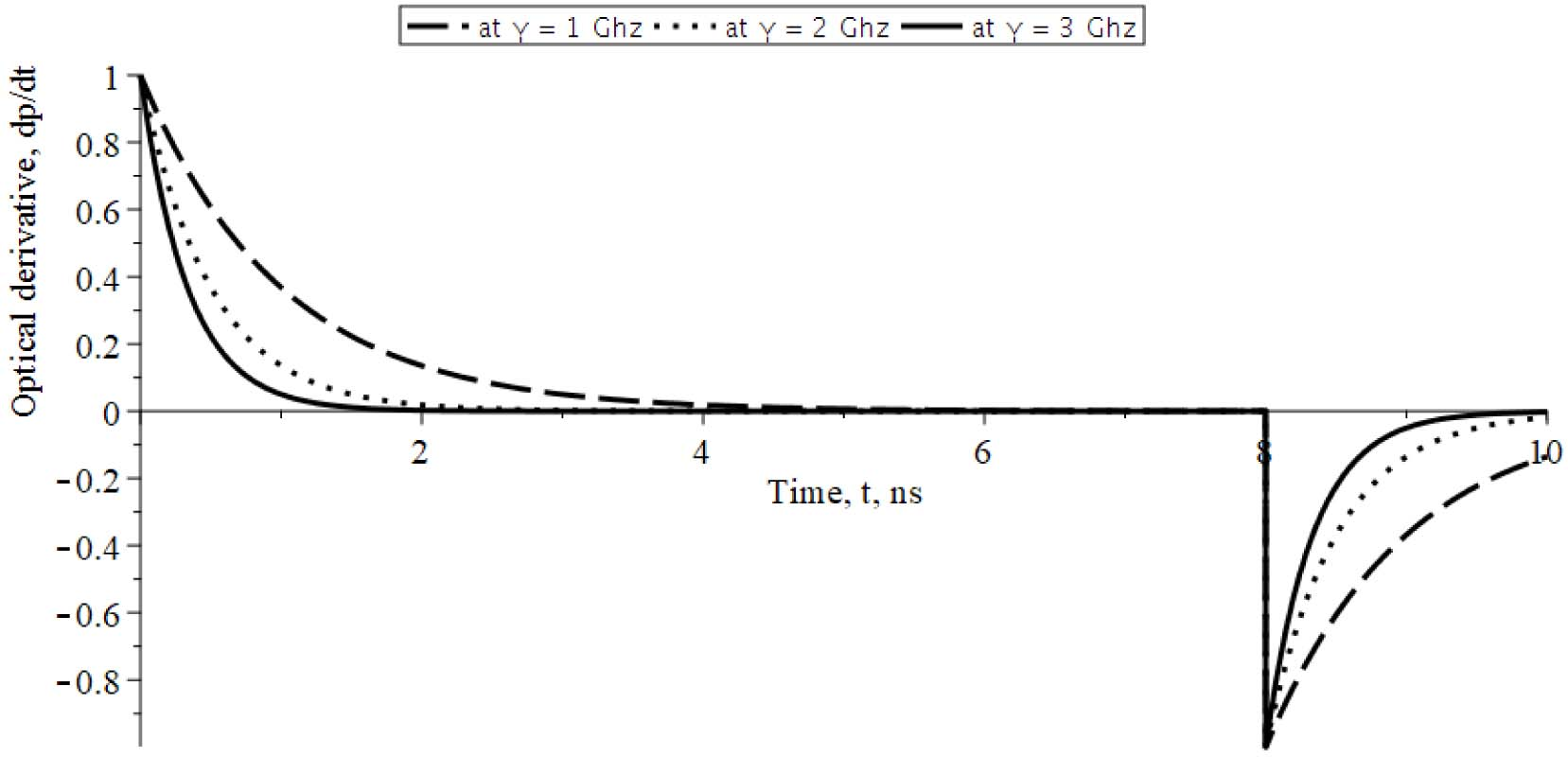As regarding the LED mode of the VCSEL, the transient behavior of the device under test in the LED mode and at different values of damping frequency is shown in Fig. 8. It is clear that, the output power varies linearly until reached a steady state value. This value is strongly depends on the damping frequency where it increases with the decrease in this damping frequency. Also the output response reaches the steady state value at earlier time with the increase of the damping frequency. The output derivative of the output as a measure of speed of the device under the LED mode is illustrated in Fig. 9. It is shown that, the device can reach the larger steady state values with a faster speed than those reach the lower steady state values. After reaching the steady state, the speed of the device is stable until the end of the time of the input pulse. The device goes to the off state with a faster speed for the larger values of damping frequency.

## IV. CONCLUSIONS

An analytical model of a Vertical Cavity Surface Emitting Laser (VCSEL) is developed in this article. The device is excited by a rectangular pulse with different duration widths. This is an effective scheme to utilize this type of devices for a high speed and long haul optical systems. The model is based on the transfer function of VCSEL. The time response and output derivative as a speed measure is analyzed at different pulse widths. The device gives a peak optical power at a very short time of the pulse duration and reaches to the steady state before turning off after the pulse duration end. The device exhibits a faster speed for larger resonance frequency near the peak power region, while it exhibits a faster speed for lower resonance frequency near the minimum power region. The dynamic response of this device under other pulse profiles is planned as a future extension of this work. This type of models can be exploited in the high speed data rate optical fiber communication systems.

### REFERENCES

1
Cheng Lu I., 2015., Very High Bit-Rate Distance Product Using High-Power Single-Mode 850 nm VCSEL with Discrete Multi-Tone Modulation Formats Through OM4 Multi-Mode Fiber, IEEE J. of Sel. Topics in Quantum Electronics, Vol. 21, No. 6, pp. 17010092
Shi J. W., Weng W. C., Kuo F. M., Yang Y.-J., Pinches S., Geen M., 2010, High-Performance Zn-Diffusion 850-nm Vertical-Cavity Surface- Emitting Lasers With Strained InAlGaAs Multiple Quantum Wells, Photonics Journal IEEE, Vol. 2, pp. 960-9663
Shi J.-W., Wei Z.-R., Chi K.-L., Jiang J.-W., Wun J.-M., Lu I., 2013, Single-Mode, High-Speed, and High-Power Vertical-Cavity SurfaceEmitting Lasers at 850 nm for Short to Medium Reach (2 km) Optical Interconnects, Journal of Lightwave Technology, Vol. 31, pp. 4037-40444
Anjin Lju, Philip Wolf, James A. Lott, 2019, Vertical-cavity surface-emitting lasers for data communication and sensing, J. of Photonics Research, Vol. 75
Murali Krishna K., Ganesh Madhan M., Ashok P., September 2020, Study of Gain Switching in Vertical Cavity Surface Emitting Laser under Different Electrical Pulse Inputs, Journal of Defence Science, Vol. 70, No. 5, pp. 538-5416
Seurin J.-F., Zhou D., Xu G., Miglo A., Li D., Chen T., Guo B., Ghosh C., 2016, High- efficiency VCSEL arrays for illumination and sensing in consumer applications, Proc. SPIE 9766, Vol. 97660D7
Grabherr M., 2015, New applications boost VCSEL quantities: recent developments at Philips, Proc. SPIE 9381, pp. 9381028
Ming-Jun Li, Fellow , IEEE , Kangmei Li, Xin Chen, Snigdharaj K. Mishra, Adrian A. Juarez, Jason E. Hurley, Jeffery S. Stone, Chia-Hsuan Wang, Hao-Tien Cheng, Chao-Hsin Wu, 2021., Single-Mode VCSEL Transmission for Short Reach Communications, Journal of Lightwave Technology, Vol. 39, No. 4, pp. 159
Yixin Cao, 2020, Development of Vertical Cavity Surface Emitting Laser Modulation for Data Communication, Journal of Physics: Conference Series, pp. 165310
Chang Y.-C., Wang C., Johansson L., Coldren L., 2006, High-efficiency, high-speed VCSELs with deep oxidation layers, Electron. Lett., Vol. 42, No. 22, pp. 1281-128211
Azuchi M., Jikutani N., Arai M., Kondo T., Koyama F., 2003, Multioxide layer vertical-cavity surface-emitting lasers with improved modulation bandwidth, in Proc. Conf. Lasers Electro-Opt., Vol. 1, pp. 16312
Moser P., Hofmann W., Wolf P., Lott J. A., Larisch G., Payusov A., Ledentsov N. N., Bimberg D., 2011, 81 fJ/bit energy-to-data ratio of 850 nm vertical-cavity surface-emitting lasers for optical interconnects, J. Appl. Phys. Lett., Vol. 98, pp. 23110613
Luo J. W., Liu* K., Wei Q., Huang Y. Q., Duan X. F., Wang Q., Ren X. M., Cai S. W., 2019, Integrated Chip for Simultaneous Transmission and Reception in Optical Communication Links, J. Acta Optica Sinica, Vol. 39, No. 8, pp. 64-7014
Petter Westbergh, Johan S. Gustavsson, Asa Haglund, Mats Skold, Andrew Joel, 2009, High-Speed, Low-Current-Density 850 nm VCSELs, IEEE Journal of Selected Topics in Quantum Electronics, Vol. 15, No. 315
Wissam Hamad, Stefan Wanckel, 2016, Small-Signal Analysis of Ultra High-Speed Multi-Mode VCSELs, IEEE Journal of Quantum Electronics, Vol. 52, No. 7, pp. 1-12## Author

Shaban M. Eladl, born in 1970, received the BS and MS degrees in Electronics and Communications (Electrical Engineering) from Faculty of Electronic Engineering, Menoufia University, Egypt in 1993 and 1999, respectively.

In 2004, he received PhD degree in Electronics and Communications (Electrical Engineering) from Faculty of Engineering, Al-Azhar University, Cairo, Egypt.

Since 1997, he has been with the Egyptian Atomic Energy Authority (EAEA), Cairo, Egypt.

Since 2009-2020, he has been with the Electrical Engineering Department, Faculty of Engi-neering, Jazan University, Jazan, KSA.

He is currently working as Associate Professor in Radiation Engineering Department, Egyptian Atomic Energy Authority (EAEA), Cairo, Egypt.

His main areas of research interest are Optoelectronics, optical communications, signal pro-cessing simulation and modelling.

Ashraf Nasr was born in (July 1968) Egypt, he earned Bachelor's degree and Master degree in electrical communications from faculty of electronic engineering, Mnuofia Univ. in 1993, and 1997 respectively.

He get PhD degree in Nano devices for IR and ionizing radiation detection from Faculty of engineering, Al-Azhar Univ. into Jan. 2004.

From he graduated till now, he specialized into optical communications, networks and optoelectronic devices.

Especially, the nanotechnology devices such as quantum dots, wires, wells, and carbon nanotube and its application into electronic and ionizing radiation fields.

He had published more fifty international scientific papers, review articles and chapter books.

He worked full time at college of computer (COE), Qassim Univ. (QU) to ten years ago.

He participated into preparing under graduate, higher programs, ABET accreditation, and other teaching activates.

From 2016, he was full professor.

Now he is the head of Radiation Engineering Dept. NCRRT, Egyptian Atomic Energy Authority (EAEA).

He welcome to any cooperation in his field of interest.

Abdelhameed Sharaf born in 1974 in Mansoura, Egypt.

He received the B.Sc. Degree in electronics engi-neering from Mansoura University, El Mansoura, Egypt in 1997, the M.Sc. degree in electronics engi-neering from Cairo University, Cairo, Egypt in 2006, and the Ph.D. Degree in electrical engineering from Cairo University, Cairo, Egypt, in 2011.

His research and teaching interests include design and application of Microelectromechanical systems (MEMS), Nanomaterials characterization and applications, Elec-tronic sensors and applications.

Abdelhameed currently serves as an associate Professor of radiation engineering, National center for radiation research and technology, Egyptian atomic energy authority.